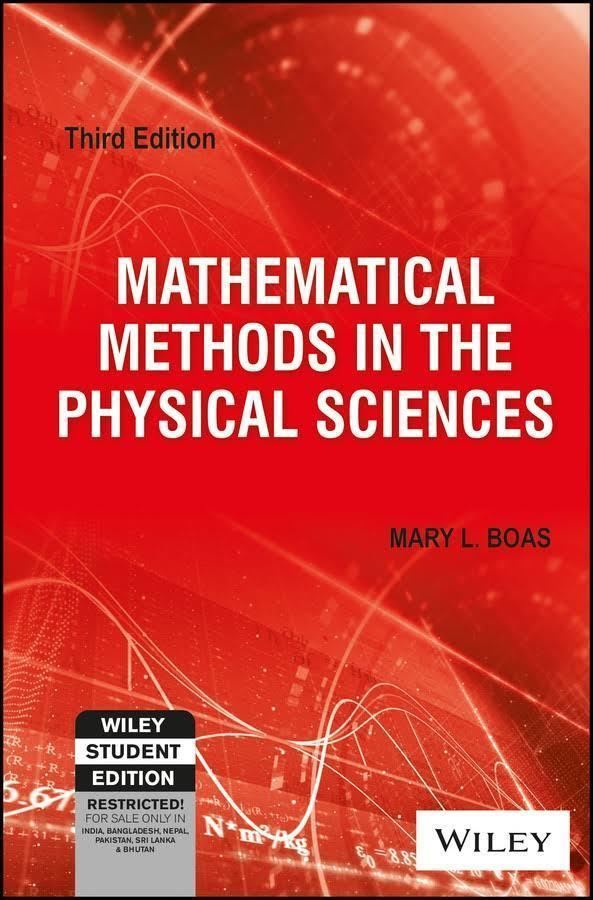# Mathematical Methods in the Physical Sciences

Updated on
Covid-19
8.2
1 Ratings
 10 0 9 0 8 1 7 0 6 0 5 0 4 0 3 0 2 0 1 0
Rate This

Rate This

Country  USA
Media type  Print
Originally published  1966
Publisher  John Wiley & Sons
Editor

Publication date  1966
ISBN  978-0-471-19826-0
Author
Subject  Applied mathematicsSimilar  Applied mathematics books, Mathematical physics books

Mathematical Methods in the Physical Sciences is a 1966 textbook by mathematician Mary L. Boas intended to develop skills in mathematical problem solving needed for junior to senior-graduate courses in engineering, physics, and chemistry. The book provides a comprehensive survey of analytic techniques and provides careful statements of important theorems while omitting most detailed proofs. Each section contains a large number of problems, with selected answers. Numerical computational approaches using computers are outside the scope of the book.

## Contents

The book, now in its third edition, is still widely used in university classrooms and is frequently cited in other textbooks and scientific papers.

## Chapters

1. Infinite series, power series
2. Complex numbers
3. Linear algebra
4. Partial differentiation
5. Multiple integrals
6. Vector analysis
7. Fourier series and transforms
8. Ordinary differential equations
9. Calculus of variations
10. Tensor analysis
11. Special functions
12. Series solution of differential equations; Legendre, Bessel, Hermite, and Laguerre functions
13. Partial differential equations
14. Functions of a complex variable
15. Integral transforms
16. Probability and statistics

## References

Similar Topics
Kid Blue
Zielen van Napels
Chris Dunk
Topics